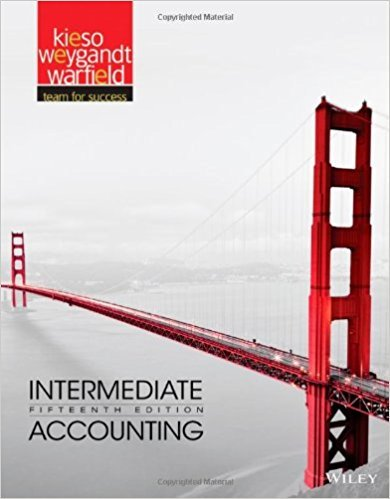×
×

# Solutions for Chapter 13: Intermediate Accounting 15th Edition## Full solutions for Intermediate Accounting | 15th Edition

ISBN: 9781118147290Solutions for Chapter 13

Solutions for Chapter 13
4 5 0 352 Reviews
19
3
##### ISBN: 9781118147290

This textbook survival guide was created for the textbook: Intermediate Accounting, edition: 15. Chapter 13 includes 31 full step-by-step solutions. This expansive textbook survival guide covers the following chapters and their solutions. Since 31 problems in chapter 13 have been answered, more than 11045 students have viewed full step-by-step solutions from this chapter. Intermediate Accounting was written by and is associated to the ISBN: 9781118147290.

Key Business Terms and definitions covered in this textbook
• Arrow’s impossibility theorem

a mathematical result showing that, under certain assumed conditions, there is no scheme for aggregating individual preferences into a valid set of social preferences

• average tax rate

total taxes paid divided by total income

• demand curve

a graph of the relationship between the price of a good and the quantity demanded

• diminishing marginal product

the property whereby the marginal product of an input declines as the quantity of the input increases

• diminishing marginal product

the property whereby the marginal product of an input declines as the quantity of the input increases

• efficiency wages

above-equilibrium wages paid by firms to increase worker productivity

• explicit costs

input costs that require an outlay of money by the firm

• incentive

something that induces a person to act

• liquidity

the ease with which an asset can be converted into the economy’s medium of exchange

• lump-sum tax

a tax that is the same amount for every person

• marginal cost

the increase in total cost that arises from an extra unit of production

• market economy

an economy that allocates resources through the decentralized decisions of many firms and households as they interact in markets for goods and services

• natural monopoly

a monopoly that arises because a single firm can supply a good or service to an entire market at a smaller cost than could two or more firms

• negative income tax

a tax system that collects revenue from high-income households and gives subsidies to lowincome households

• negative income tax

a tax system that collects revenue from high-income households and gives subsidies to lowincome households

• public saving

the tax revenue that the government has left after paying for its spending

• quantity equation

the equation M × V = P × Y, which relates the quantity of money, the velocity of money, and the dollar value of the economy’s output of goods and services

• theory of liquidity preference

Keynes’s theory that the interest rate adjusts to bring money supply and money demand into balance

an excess of imports over exports